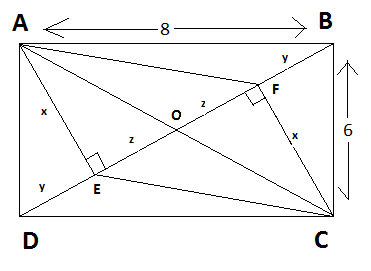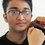# Stubborn RectangleABCD is a rectengle.$$AE \perp BD$$ and $$CF \perp BD$$.Let AE=x unit,ED=BF=y unit and OE=OF=z unit.AB=$$8$$ unit;BC=$$6$$ unit.What is the value of $$\left \lfloor (x+y+z)^2 \right \rfloor$$Note by Fazla Rabbi
7 years ago

This discussion board is a place to discuss our Daily Challenges and the math and science related to those challenges. Explanations are more than just a solution — they should explain the steps and thinking strategies that you used to obtain the solution. Comments should further the discussion of math and science.

When posting on Brilliant:

• Use the emojis to react to an explanation, whether you're congratulating a job well done , or just really confused .
• Ask specific questions about the challenge or the steps in somebody's explanation. Well-posed questions can add a lot to the discussion, but posting "I don't understand!" doesn't help anyone.
• Try to contribute something new to the discussion, whether it is an extension, generalization or other idea related to the challenge.

MarkdownAppears as
*italics* or _italics_ italics
**bold** or __bold__ bold
- bulleted- list
• bulleted
• list
1. numbered2. list
1. numbered
2. list
Note: you must add a full line of space before and after lists for them to show up correctly
paragraph 1paragraph 2

paragraph 1

paragraph 2

[example link](https://brilliant.org)example link
> This is a quote
This is a quote
    # I indented these lines
# 4 spaces, and now they show
# up as a code block.

print "hello world"
# I indented these lines
# 4 spaces, and now they show
# up as a code block.

print "hello world"
MathAppears as
Remember to wrap math in $$ ... $$ or $ ... $ to ensure proper formatting.
2 \times 3 $2 \times 3$
2^{34} $2^{34}$
a_{i-1} $a_{i-1}$
\frac{2}{3} $\frac{2}{3}$
\sqrt{2} $\sqrt{2}$
\sum_{i=1}^3 $\sum_{i=1}^3$
\sin \theta $\sin \theta$
\boxed{123} $\boxed{123}$

Sort by:

Well this problem looks simple First we see by Pythagoras theorem in triangle $ADB$ that $BD=10$ So We conclude that $y+z=10/2=5$ Now area of triangle $ADB$ is 24 So $24=\frac{1}{2}×BD×AE$ i.e.$24=\frac{1}{2}×10×AE$ i.e. $AE=\frac{48}{10}$ So we get what we desire

- 7 years ago

The diagonals of a rectangle bisect each other. Furthermore, by Pythagorean's theorem, $AC = 10$, so $AO = DO = 5$. Using these same results, we get

$y + z = 5$

$x^2 + z^2 = 25$

$x^2 + y^2 = 36$

Subtracting the last two equations we get $y^2 - z^2 = 11$, so $(y - z)(y+z) = 11$. Substituting the first equation, we get $y-z = \frac{11}{5}$. Doing a linear combination with $y+z = 5$ we get $2y = \frac{36}{5}$ so $y = \frac{18}{5}$ and $z = \frac{7}{5}$. Using one of the equations to find $x$ we get that $(x+y+z)^2 = (\frac{49}{5})^2 = \frac{2401}{25}$, and the greatest integer less than or equal to that is $96$.

Motivations in this problem: Nothing really too fancy, just finding what we're given using pythagorean's theorem (which should be obvious given the right angles and side lengths of $6$ and $8$) and then solving a system of equations.

- 7 years ago

Wow nice approach. Thanks for one more method.

- 7 years ago

(24/5 + 5)^2

- 7 years ago

I thnk answer is 96.04 In triangle ABC = AB^2 + BC^2 = AC^2 64 + 36 = 100 AC = 10 AC = BD = 10 then 2(y+z) = 10 y+z = 5 z = 5-y (1) AD = BC= 6 then AD^2 = DE2 + AE2 36 = X2 + Y2 (2) In Triangle AOE and COF OE = OF ANGLE AEO = ANGLE CFO ANGLE AOE = ANGLE COF TRIANGLE AOE CONGRUENT TO TRIANGLE COF THEN AO = OC so AC= 10 then AO = 5 Also In Triangle AOE AO2 = AE2+OE2 25 = x2 + z2 (3) 25 = x2 + (y-5)2 25 = x2 + y2 + 25 - 10y 0 = x2+y2 - 10y(4) subtracting 4 from 2 we get 10y = 36 y = 3.6 from (1) we get z = 1.4 putting value of z in 3rd equation we get 25 = x2 + 1.96 x2 = 23.04 x = 4.8 x+y+z = 1.4+3.6+4.8 = 9.8 (x+y+z)2 = (9.8)2 = 96 .04

- 7 years ago

Ans is 96.04

- 7 years ago

96.04

- 7 years ago

(9.8)^2 means 96.04 is the answer.

- 7 years ago

96.04

- 7 years ago

answer is 96.04 as here BO=5cm and area boc=68/4=12=1/25*x this means x=24/5=4.8 so value=(5+4.8)square=(9.8)square=96.04

- 7 years ago

96.04 is the ans

- 7 years ago

96.04

- 7 years ago

96.04

- 7 years ago

96.04

- 7 years ago

96.04

- 7 years ago

correct ans : 96.04

- 7 years ago

- 7 years ago

In triangle ADB, by using Pythagoras theorem, we can say that BD=10.Area of triangle ABD=24=1/2(10)(AE), thus, AE=x=4.8.And as O is the mid-point of BD, DO=z+y=5. Therefore, (x+y+z)^2=(4.8+5)^2=96.04

(x+y+z)power2 =100

- 7 years ago

true

- 7 years ago

2z+2y=10 & (z+y=5) _(1) ((2z+y)^2)+(x^2)=64 4z^2+y^2+4yz+x^2=64 ((5-z)^2 -z^2=11 25+z^2-10z=11 z=7/5 y=(18/5) x=(24/5) z+y+x=(7/5)+(18/5)+(24/5)) z+x+y=10

(z+x+y)^2=100 unit

- 7 years ago

true

- 7 years ago

SOLVE THIS PROBLEM

- 7 years ago

SOLVE THIS PROBLEM

- 7 years ago

96.04

- 7 years ago

96.04

- 7 years ago

96.04

- 7 years ago

96.04

- 7 years ago

96.04

- 7 years ago

96.04

- 7 years ago

121

- 6 years, 10 months ago

- 7 years ago

121

- 7 years ago

- 7 years ago

2401/25

- 7 years ago

ans is 84

- 7 years ago